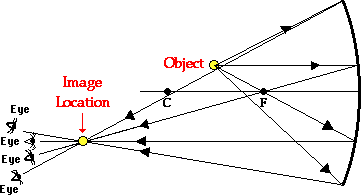Home » Posts tagged 'object'

# Tag Archives: object

### Subscribe to this site by email## Weight and inertia on moon

Say True or False; An object on the Moon weights 1/6 of what it weights on the Earth. Therefore, the object has less inertia on the Moon.( Justify your answer)

Inertia depends on mass and not on weight. The weight on moon is less not because of a decrease in mass, but due to the decrease in the acceleration due to gravity on the surface of moon.

Inertia depends on mass and there is no change in mass when a body is taken to moon. Therefore the inertia of a body is same on moon as that on earth or anywhere else in the universe, even in a gravity free space.

## Is it true that a person touching a high power line get struck with the line?

Is it true that a person touching a high power line get struck with the line?

Ya, This is due to the strong contraction of the muscles making the person not at all able to release the wire or object from which he got the shock. Not due to any type of attraction arising out of electricity.

## Buoyant force

What is buoyant force? I’m unable to understand practically from book. please give vast and practical explanation.. (Raj asked)

Whenever a body is immersed in a fluid, say water, it experiences an upward force acting on it. The upward force exerted by a fluid on an object immersed in it is called Buoyant force.When an object is immersed, it displaces the fluid already present there. The upward force (buoyant force) is the result of the fluid’s attempt to reoccupy the space.

If the object placed in a fluid is denser than the fluid, it will sink. Though it experiences an upthrust, its weight will be more than the upthrust.

The upthrust is equal to the weight of liquid displaced by the immersed part of the body.

If the object is of less density than the fluid, it will come up and float.

For a floating body, the net weight is equal to zero, as the upthrust balances the actual weight of the body.

## Angle between velocity and acceleration

Discuss a circumstance in which angle between velocity and acceleration is less than 90.

Discuss a circumstance in which angle between velocity and acceleration lies batween 180 and 90.

In projectile motion when the object is moving up, the angle between velocity and acceleration (due to gravity g) is between 90 degree and 180 degree.

during the downward motion of a projectile, the angle between velocity and acceleration is between 0 and 90 degree.

## Displacement and acceleration in real life

HOW DISPLACEMENT,ACCELERATION ACTS IN REAL LIFE PLZ GIVE SOME EXAMPLE.

(The question was posted by Chandrima )

As you know, the displacement is the shortest distance between two points ; the initial point and the final point. The displacement is independent of the path followed by the object and this itself is the beauty of the concept of displacement. We may take different paths to reach a destination, and the length of the path may be different, but all the paths result in the same displacement.

## Optics MCQ – image formation by concave mirror

I am having trouble with a problem.

Q. In an experiment to determine the focal length (f) of a concave mirror by the u–v method, a student places the object pin A on the principal axis at a distance x from the pole P. The student looks at the pin and its inverted image from a
distance keeping his/her eye in line with PA. When the student shifts his/her eye towards left, the image appears to the right of the object pin. Then

(A) x < f

(B) f < x < 2f

(C) x = 2f

(D) x > 2fEXPLANATION

The image and the object will move together at 2f

The one closer to the eye will move faster

In the question, the situation occurs when the image is closer to the eye and this happens when the object is between f and 2f

## A numerical Problem from optics

When an object is kept at a distance of 60cm from a concave mirror, the magnification is 1/2. Where should the object be placed to get magnification of 1/3??

Ans:

In the first part, u=-60, m=(-)1/2 implies v=-30 cm (Since the image must be real as concave lens form virtual image of bigger size only)

we get f = –20 cm

In II case

m=-1/3

v=u/3

f=-20cm

we get u=4f=4 x (-20)=-80 cm

### Hits so far @ AskPhysics

• 2,239,500 hits